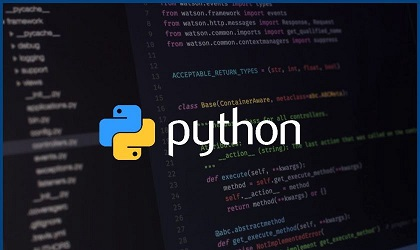# How to Iterate Through List of Dictionaries in Python

Python is a powerful language that allows you to work with different types of data structures. Sometimes you may need to iterate through list of dictionaries in python. In this article, we will look at different ways to iterate through list of dictionaries in Python.

## How to Iterate Through List of Dictionaries in Python

There are multiple ways to iterate through list of dictionaries in python.

Basically, you need to use a loop inside another loop. The outer loop, iterates through each dictionary, while the inner loop iterates through the individual elements of each dictionary. There are different ways to do this.

You can simply iterate over the range of length of list.

```dlist = [{'a': 1}, {'b': 3}, {'c': 5}]
for index in range(len(dList)):
for key in dList[index]:
print(dList[index][key])```

In the outer loop, we use range and length function to create a list that we can iterate through. We use the index value to get each dictionary. In the inner loop, we use key variable to iterate through the current dictionary.

Alternatively, you can lop using while loop with an index.

```dList = [{'a': 1}, {'b': 3}, {'c': 5}]
index = 0
while index < len(dList):
for key in dList[index]:
print(dList[index][key])
index += 1```

In the above code, we modify the outer loop to use while condition instead of for statement. We use len function to get the length of list of dictionaries and use the index counter to increment from 0 as long as it is less than the length of dictionary list.

You can also directly loop through the dictionary elements.

```dList = [{'a': 1}, {'b': 3}, {'c': 5}]
for dic in dList:
for key in dic:
print(dic[key])```

You can even use a shortcut that directly loops through the dict, without using len or range functions, in the outer loop. In the inner loop too, there is no need for any lookup.

If you don’t want to do any lookups, you can use the following method.

```dList = [{'a': 1}, {'b': 3}, {'c': 5}]
for dic in dList:
for val in dic.values():
print(val)```

Finally, you can also use list comprehensions as shown below to loop through list of dictionaries.

```dList = [{'a': 1}, {'b': 3}, {'c': 5}]
print(*[val for dic in dList for val in dic.values()], sep='\n')```

In this article, we have looked at different ways to loop through list of dictionaries in Python. You can use any of them as per your requirement.